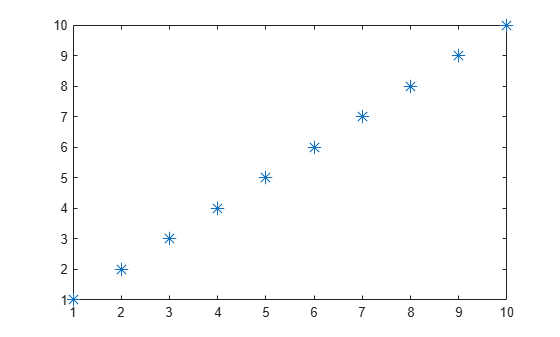# Clipping in Plots and Graphs

This example shows how MATLAB® uses clipping in plots and how to control clipping.

### What Is Clipping?

Clipping occurs when part of an object extends outside the boundaries of the axes in a plot. In MATLAB®, the part of the object that is clipped does not appear on the screen or in printed output. By default, MATLAB clips most objects at the axis limits.

### Turn Clipping Off

#### Clipping in 2-D Plots

Clipping occurs in 2-D plots. For example, MATLAB clips the sine wave in this 2-D plot.

```x = 0:pi/20:2*pi; y = sin(x); plot(x,y) xlim([0,2*pi]) ylim([-0.9 0.9])```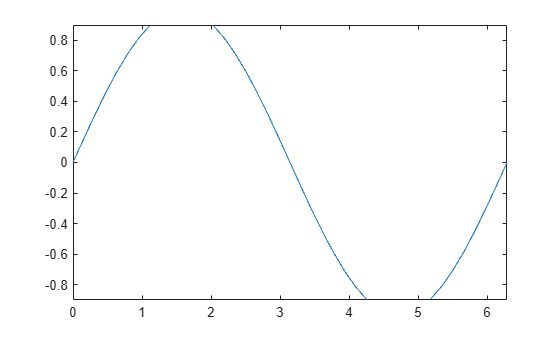You can control the clipping of the sine wave by using the axes `Clipping` property. Turn clipping `"off"` to display the entire sine wave.

```ax = gca; ax.Clipping = "off";```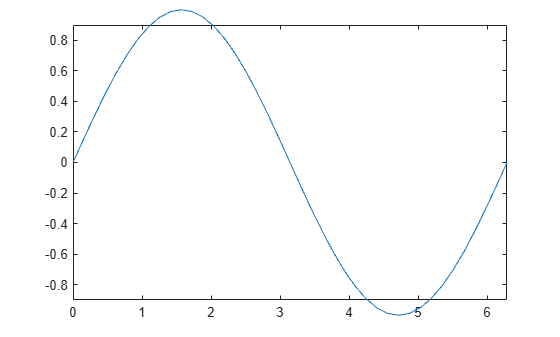#### Clipping in 3-D Plots

Clipping also occurs in 3-D plots. For example, MATLAB clips the surface in this 3-D plot.

```figure surf(peaks) zlim([-4 4])```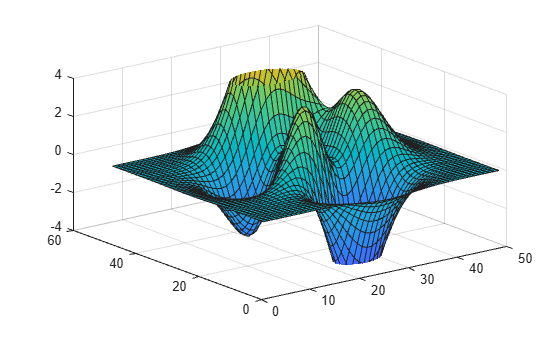You can control the clipping behavior of the axes by using the axes `Clipping` property. Turn clipping `"off"` to display the entire surface.

```ax = gca; ax.Clipping = "off";```### Control the Clipping Style

Use the `ClippingStyle` property to control the way clipping works with respect to the axes. If you set `ClippingStyle` to `"3dbox"`, then MATLAB clips objects to the volume defined by the limits of the x-, y-, and z-axes. If you set `ClippingStyle` to `"rectangle"`, then MATLAB clips objects at an imaginary rectangle drawn around the outside of the x-, y-, and z-axes. These plots show the difference between the two clipping styles.### Control Text Clipping

Objects other than axes have the `Clipping` property. MATLAB can also clip text objects at the axes plot box. For example, plot some data and add a text description. By default, the `Clipping` property for text objects is set to `"off"`, so if you zoom or pan, text can extend outside the axis limits.

```figure x = 1:10; y = -10:10; [X,Y] = meshgrid(x,y); Z = X.^2-Y.^2; surf(X,Y,Z); ylim([-20.5 1.5]) t = text(1,7,60,"example");```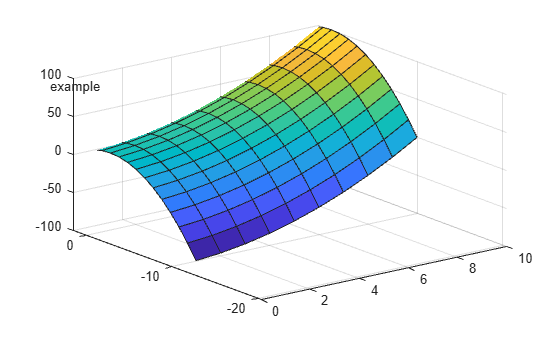If you set the text `Clipping` property to `"on"`, the full text disappears from view when the `(x,y,z)` position of the text object, also known as the anchor point, is outside the axes plot box.

`t.Clipping = "on";`If you want the text to clip at the axes plot box and show partial text, then also set the axes `ClippingStyle` property to `"rectangle"`.

```ax = gca; ax.ClippingStyle = "rectangle";```### Clipping and Markers

Clipping does not affect markers drawn at each data point as long as the data point itself is inside the x- and y-axes limits of the plot. MATLAB displays the entire marker even if it extends slightly outside the boundaries of the axes.

```p = plot(1:10,'*'); p.MarkerSize = 10; axis([1 10 1 10])```